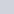Student Forums CMA Part 2 Section E: Investment Decisions E.2. Capital Analysis Analysis Methods Mock Exam 1 . Question 57. NPV calculation

# Mock Exam 1 . Question 57. NPV calculation

• This topic has 2 replies, 2 voices, and was last updated 1 year, 8 months ago byLynn Roden.
• Creator
Topic
• #140664amulour
Participant

Dear Ms. Lynn,
Mock Exam 1 Question 57:
Cash flow discounted at 20% for 5 years: \$23,000 × 2.991 = \$ 68,793
Final year excess CF discounted at 20%:
(\$41,000 − \$23,000) × 0.402 = 7,236
Doubt:
In the above, cashflow is discounted for 5 years as though there is a constant cashflow of 23000 for all 5 years.
However, since plant is depreciated fully in 4 years, there would be no depreciation shield in the 5th year, isn’t it?
So cashflow in 5th year should be \$18,000. But it is considered as \$ 20,000.
Would you kindly explain?

Viewing 2 replies - 1 through 2 (of 2 total)
• Author
Replies
• #140665amulour
Participant

Dear Ms.Lynn,

This is the question for above query.
The following information is for Questions 55-58: Kampit, Inc. is contemplating a 5-year capital
investment project with estimated revenues of \$80,000 per year and estimated cash operating expenses
of \$50,000 per year. The initial cost of the plant and equipment for the project is \$50,000, and the
company expects to sell the equipment for \$5,000 at the end of the 5th year. P&E will be fully
depreciated over 4 years on a straight-line basis for tax purposes. The project requires a working capital
investment of \$20,000 at its inception. The cost of capital for Kampit is 10%. Assume a 40% marginal
tax rate for the company.

#140681Lynn Roden
HOCK international

Hi amulour,

You are correct that the depreciation tax shield is for only 4 years. There is more than one way to calculate the present value of 4 years of 23,000 cash flows plus a fifth year of a cash flow of 41,000, and the way it is done in that answer explanation is just one of them.

We could discount 23,000 as an annuity for 4 years and the 41,000 as the present value of 1 for 5 years and we would get the same present value for the five cash flows as is calculated in the answer explanation.

Or, we could do as was done in the answer explanation and discount 23,000 of the fifth year’s cash flow as part of the annuity and discount the annuity for 5 years; and then discount the incremental amount of the fifth year’s cash flow (the amount over and above the 23,000) as the present value of 1 for 5 years.

Neither way is better, and both result in the same present value. Here is the NPV calculated as four years of an annuity plus the fifth year as a separate amount:

Four years of an annuity of 23,000 discounted at 20% is 23,000 x 2.589 = 59,547.
41,000 discounted for 5 years at 20% is 41,000 x 0.402 = 16,482.
The present value of the cash inflows is 59,547 + 16,482 = 76,029.
The NPV is 76,029 minus the initial investment of 70,000, which equals 6,029.

That is the same NPV as was calculated in the answer explanation. The only difference is that instead of summing 59,547 and 16,482 as I just did to calculate the present value of the cash inflows as 76,029, the answer explanation sums 68,793 and 7,236 to calculate the present value of the cash inflows as 76,029, the same amount.

Either way works, and neither way takes less time than the other, so they are both fine.

Lynn

• This reply was modified 1 year, 8 months ago byLynn Roden.
Viewing 2 replies - 1 through 2 (of 2 total)
• You must be logged in to reply to this topic.